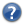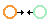# VizieRJ/A+A/282/899 626 Teff and angular diameters from IRFM (Blackwell+ 1994) ReadMe+ftp1994A&A...282..899BSimilar Catalogs 1. J/A+A/282/899/table1 Values of Log R as a function of temperature, wavelength and surface gravity (72 rows) 2. J/A+A/282/899/table2 Effect on determined effective temperatures of using Kurucz (1992) model atmospheres instead of MARCS atmospheres (4 rows) 3. J/A+A/282/899/table3 Effect on determined temperatures at J, K, L for a change in surface gravity (18 rows) 4. J/A+A/282/899/table4 Effect of A(V) on effective temperatures (6 rows) 5. J/A+A/282/899/table5 Effect of a one per cent decrease in flux at J, K, L wavelengths on temperatures (5 rows) 6. J/A+A/282/899/table6 Parameters of selected stars (80 rows) 7. J/A+A/282/899/table7 Effect of A(V) on the integrated flux (6 rows) 8. J/A+A/282/899/table8 Coefficients A,B,C in the relation temperature = A+B*(V-K)+C*(V-K)**2 (3 rows) 9. J/A+A/282/899/table9 Comparison between determined temperatures and calculated temperatures for stars which as not been detected as spectroscopic binaries (48 rows) 10. J/A+A/282/899/table10 Comparison between determined temperatures and calculated temperatures for known spectroscopic binaries (32 rows) 11. J/A+A/282/899/table11 Comparison of IRFM effective temperatures with 'adopted' temperatures of Bell and Gustafsson (19 rows) 12. J/A+A/282/899/table12 Relation between temperatures determined by Ridgway et al. and present IRFM temperatures (3 rows) 13. J/A+A/282/899/table13 Effect of A(V) on angular diameters (6 rows) 14. J/A+A/282/899/table14 Comparison between angular diameters determined by Michelson interferometry and present IRFM determinations (3 rows)

Query by Constraintsapplied on Columns (Output Order: + - )
ShowSortJoin tablesColumn  Constraint Explain   (UCD)
(ALL)recno  Record number assigned by the VizieR team. Should Not be used for identification.
(1+6)log.g [cm/s2] Surface gravity
(1+2+3+4+5+6+7+13)Teff K Effective temperature
(1)LogR1  Ratio of integrated flux to monochromatic flux lambda 1.2467 um
(1)LogR2  Ratio of integrated flux to monochromatic flux lambda 2.2135 um
(1)LogR3  Ratio of integrated flux to monochromatic flux lambda 3.7825 um
(2+5)TcJ K Temperature change for use of filter J
(2+5)TcK K Temperature change for use of filter K
(2+5)TcL K Temperature change for use of filter L
(3)Filter (char) Filter used
(3)Tc2 K Temperature change for log g=2

ALL cols

 (i)indexed column
(3)Tc3 K Temperature change for log g=3
(3)Tc4 K Temperature change for log g=4
(4+7+13)CF5  Correction factors for A(V)=0.05m
(4+7+13)CF10  Correction factors for A(V)=0.10m
(4+7+13)CF15  Correction factors for A(V)=0.15m
(4+7+13)CF20  Correction factors for A(V)=0.20m
(6)HRdata (char) Link to the Bright Star Data(6+9+10+11+14)BS  (i) BS (Bright Star catalogue) number
(6)n_BS (char) When *, known spectroscopic binary
(6)SpType (char) Spectral class
(6)A(V) mag Interstellar extinction
(6)V mag Visual magnitude
(6)B-V mag B-V color

ALL cols

 (i)indexed column
(6+12)V-K mag V-K color
(6)Fe/H  (n) []? Metallicity
(6)FT 10-9W/m2 Total flux
(6)D mas Angular diameter
(8)Type (char) Kind of star
(8)A  Coefficient A
(8)B  Coefficient B
(8)C  Coefficient C
(9+10)Tm K Measured temperature
(9+10)Tc K Calculated temperature (Note 1)
(9+10)R % Residual (measured - calculated temperature)
(11)Tbg K Adopted temperature of Bell & Gustafsson (Note 1)
(11+12)Tirfm K IRFM (Infrared Flux Method) effective temperature

ALL cols

 (n) indicates a possible blank or NULL column (i)indexed column
(11)Tirfm-Tbg % Temperature differences Tirfm - Tbg
(12)Tr K Adopted temperature of Ridgway et al. (Note 1)
(12)Tirfm-Tr K Temperature differences Tirfm - Tr
(12)DT/T % Temperature differences Tirfm - Tr percent
 Adapt form Display your selection only Reset to default columnsDisplay UCD1+    UCD1